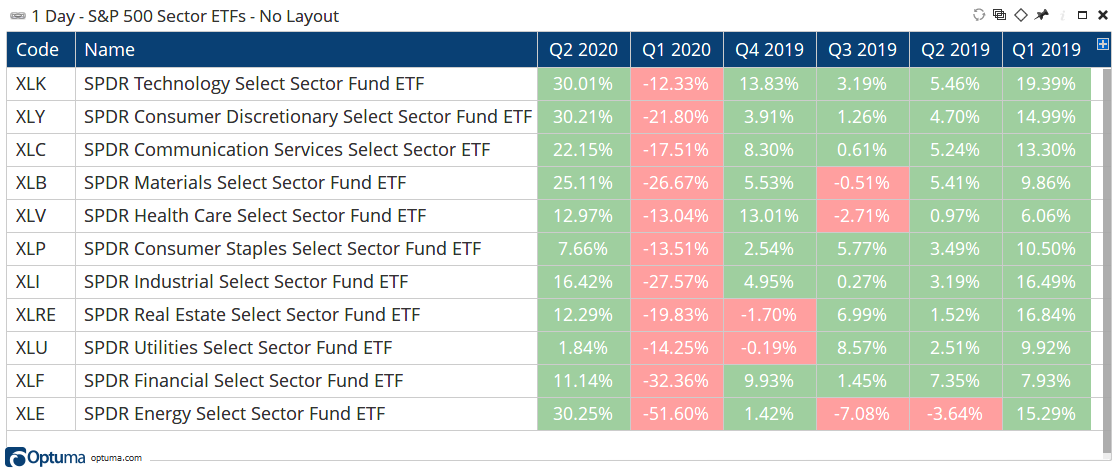# DAYNUM(), MONTHNUM(), and YEARNUM()

Author: Optuma Team Last updated: Sep 3, 2020 22:40

## Overview

These functions returns integers for their respective values:

`DAYNUM()` will show the day of the month, ranging from 1 to 31.

`MONTHNUM()` shows the month as a value between 1 and 12.

`YEARNUM()` will show the year eg 2020.

### Example

Example showing 2020 2nd quarter performance using the `MONTHNUM()` and `YEARNUM()` functions:

``````//Get quarter end for June
M1 = MONTHNUM() == 6 and YEARNUM() == 2020;

//Get quarter end for March
M2 = MONTHNUM() == 3 and YEARNUM() == 2020;

//Get closing values for above dates
V1 = VALUEWHEN(M1);
V2 = VALUEWHEN(M2);

//Calculate % change
((V1/V2)-1)
``````